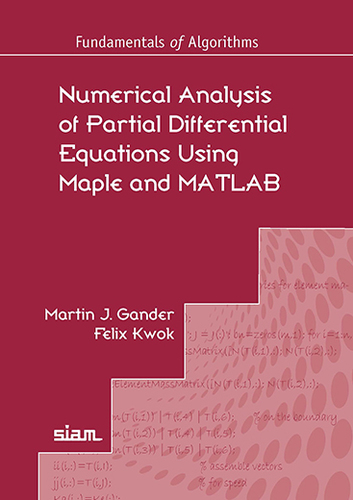Numerical Analysis of Partial Differential Equations Using Maple and MATLAB Martin J. Gander, Felix Kwok Published by SIAM
Home Summary Content Programs Authors

 Select: Home Summary Content Programs Authors
 :: Purpose and Summary Equal emphasis on theory and numerical algorithms Our book provides an elementary yet comprehensive introduction to the numerical solution of partial differential equations (PDEs). Used to model important phenomena, such as the heating of apartments and the behavior of electromagnetic waves, these equations have applications in engineering and the life sciences, and most can only be solved approximately using computers. We provide detailed descriptions of the four major classes of discretization methods for PDEs (finite difference method, finite volume method, spectral method, and finite element method) and runnable MATLAB code for each of the discretization methods and exercises. We also give self-contained convergence proofs for each method using the tools and techniques required for the general convergence analysis but adapted to the simplest setting to keep the presentation clear and complete. This book is intended for advanced undergraduate and early graduate students in numerical analysis and scientific computing and researchers in related fields. It is appropriate for a course on numerical methods for partial differential equations.

Home Summary Content Programs Authors